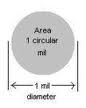# The Circular MilThe circular mil is a unit of area which is used to specify the size of wires.  The mil is a thousandth of an inch which corresponds to 0.0254mm.  The circular mil is the area of a circle whose diameter is 1 mil.  The mil is also known as thou (thousands of an inch).

The circular mil is used as the area of the circle can be calculated without the use of Pi.

Thus the cross-sectional  Area of the circle wire = D2
where D is the diamter of the circle in mils.

Larger wire sizes are rated in MCM or Thousand circular Mils.  If the conductor cross section is square shaped, the size will be indicated in Square mils.

Square Mils = π/4 * D2